## MP Board Class 9th Maths Solutions Chapter 6 Lines and Angles Ex 6.2

Question 1.
In Fig. given below, find the values of x and y and then showthat AB ∥ CD.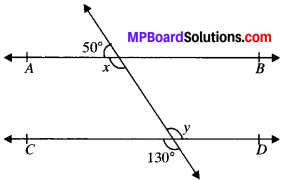Solution:
∠y = 130° (VOA’s)
x + 50° = 180° (LPA’)
∴ x = 180° – 50° = 130°
∠x and ∠y are alternate interior angles and are equal AB ∥ CD

Question 2.
In Fig. given below, if AB ∥ CD, CD ∥ EF and y : z = 3 : 7, find x.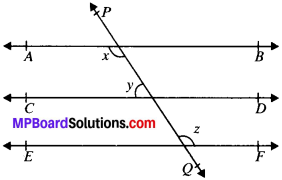Solution:
Given
AB ∥ CD
CD ∥ EF
y : z = 3 : 7
∴ y = 3k
and z = 7k
To find, x
Solution:
AB ∥ EF and PQ is the transversal
x = z (A. I.A’s)
AB ∥ CD and PQ is the transversal
∴ x + y = 180° (C.I.A.’s)
z + y = 180° (∴ x = z)
7k + 3k = 180°
∴ k = 18°
y = 3 x 18° = 54°
2 = 7 x 18° = 126°
x = z = 126°

Question 3.
In Fig. given below, if AS ∥ CD, EF ⊥ CD and ∠GED = 126°, find ∠AGE, ∠GEF and ∠FGE.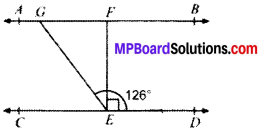Solution:
Given
AB ∥ CD
EF ⊥ CD
To find
∠AGE, ∠GEF, ∠FGE
AB ∥ CD and GE is the transversal
∴ ∠AGE = ∠GED = 126°
∠AGE + ∠FGE = 180°
126° + ∠FGE = 180°
∠FGE = 54°
∠GED = ∠GEF + ∠FED
126° = ∠GEF + 90°
126° – 90° = ∠GEF
∠GEF = 36°Question 4.
In Fig. given below, if PQ ∥ ST, ∠PQR = 110° and ∠RST = 130°, find ∠QRS.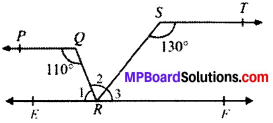Solution:
Given
PQ ∥ ST
∠PQR = 110°; ∠TSR = 130°
To find
∠QRS Construction.
Draw a line EF ∥ PQ passing through point R.
Calculation. PQ ∥ EF (by construction)
PQ ∥ ST (Given)
EF ∥ ST (lines ∥ to the same line are parallel to each other)
PQ ∥ EF and QR is the transversal
110° + ∠1 = 180° (CIA’s)
∴ ∠1 = 70°
ST ∥ EF and SR is the transversal
130° + ∠3 = 180° (CIA’s)
∠3 = 50°
∠1 + ∠2 + ∠3 = 180° (angles on the same line)
70° + ∠2 + 50° = 180°
∠2 = 180° – 120°
∴ ∠QRS = 60°

Question 5.
In Fig. given below, if AB ∥ CD, ∠APQ = 50° and ∠PRD = 127°, find x and y.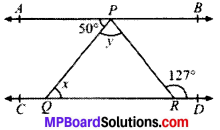Given
AB ∥ CD
∠APQ = 50°; ∠PRD = 127°
To find, x and y
Calculation:
AB ∥ CD and PQ is the transversal
∠APQ = x = 50° (AIA’s)
y + 50° = 127°
∴ y = 77°

Question 6.
In Fig. given below, PQ and RS are two mirrors placed parallel to each other. An incident ray AB strikes the mirror PQ at B, the reflected ray moves along the path BC and strikes the mirror RS at C and again reflects back along CD. Prove that AB ∥ CD.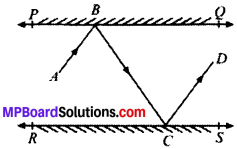Solution:
Given
PQ ∥ RS
To prove:
AB ∥ CD
Construction:
Draw MB and NC normals at point B and C respectively.
Proof:
∠1 = ∠2
(Angle of incident = Angle of reflection)
and ∠3 = ∠4
∠ABC = 2∠2 and ∠BCD = 2∠3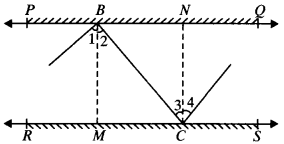MB ⊥ PQ and NC ⊥ RS
MB ∥ CN (Lines perpendicular to two parallel lines are parallel to each other)
MB ∥ CN and BC is the transversal
∠2 = ∠3 (AIA’s)
⇒ 2∠2 = 2∠3
(on multiplying both sides by 2)
⇒ ∠ABC = ∠BCD
∠ABC and ∠BCD are alternate interior angles and are equal AB ∥ CDAngle Sum Property of a Triangle:

1. Triangle:
A plane figure bounded by three line segments in a plane is called a triangle. A triangle has six parts –

• Three sides – AB, BC and AC
• Three angles – ∠A, ∠B and ∠C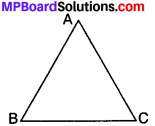2. Median:
The median of a ∆ corresponding to a particular side is the line segment joining the midpoint of that side to its opposite vertex. In Fig., D, E and F are the midpoints of sides BC, AC and AB respectively. AD, BE and CF are the medians.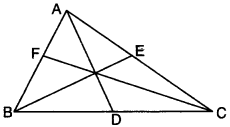3. Incentre of a triangle:
The incentre of a ∆ is the point of intersection of angle bisectors of the triangle. In Fig., OA, OB and OC are the angle bisectors of ∠A, ∠B and ∠C respectively. These angle bisectors intersect at point O. Therefore, point O is the incentre of ∆ABC.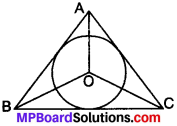4. Circumcenter of a triangle:
The circumcenter of a triangle is the point of intersection of the perpendicular bisectors of the sides of the triangle. In Fig., O is the circumcenter of ∆ABC where the perpendicular bisectors of AB, BC and AC intersect.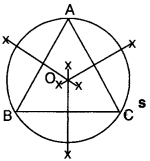Theorem 1.
The sum of the angles of a triangle is 180°.
Given
ABC is a ∆ in which ∠1, ∠2 and ∠3 are angles.
To prove:
∠1 + ∠2 + ∠3 = 180°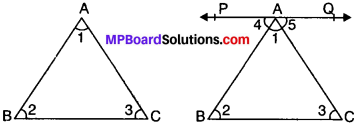Construction:
Draw a line PQ passing through point A parallel to BC.
Proof:
PQ is a line.
∠4 + ∠1 + ∠5 = 180° (angles on the same line) …..(i)
PQ ∥ BC and AB is the transversal
∴ ∠4 = ∠2 (AIA’s) …..(ii)
PQ ∥ BC and AC is the transversal
∴ ∠5 = ∠3 (AIA’s) …..(iii)
Putting ∠4 = ∠2 and ∠5 = ∠3 in (i), we get
∠2 + ∠1 + ∠3 = 180°
∴ ∠1 + ∠2 + ∠3 = 180°. ProvedExterior Angle Property:

Theorem 2.
If a side of a triangle is produced, then the exterior angle so formed is equal to the sum of the two opposite interior angles. This is also known as exterior angle property (EAP).
Given
ABC is a ∆ in which side BC is produced to point D.
To prove. ∠4 = ∠1 + ∠2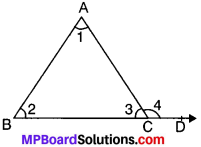Proof:
In ∆ABC,
∠1 + ∠2 + ∠3 = 180° (ASP)
∠3 + ∠4 = 180° (LPA)
From (i) and (ii), we get
∠1 + ∠2 + ∠3 = ∠3,+ ∠4
∠1 + ∠2 = ∠4. Proved

Corollary

Theorem 3.
An exterior angle of a triangle is greater than either of the opposite interior angles.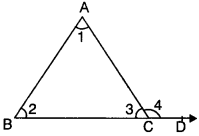Given
In ∆ABC
∠4 = ∠1 + ∠2 (EAP)
∠4 > ∠1 and ∠4 > ∠2
Hence an exterior angle of A is greater than either of the opposite interior angle.

Lines And Angles:

Example 1.
In the Fig., AB divides ∠DAC in the ratio 1 : 3 and AB = DB. Find the value of y.
Solution:
Let ∠DAB = x and ∠BAC = 3x
In ∆ABD
AB = BD
(∴ In a ∆ angles opposite to equal sides are equal)
∠ABC = x + x = 2x
(In a ∆ Exterior angle is equal to sum of two opp. interior angles) In ∆ABC
2x + 3x + y = 180° (ASP)
5x + y = 180° …..(i)
x + y = 180° (ASP)
y = 180° – X …..(ii)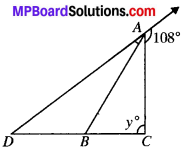Putting y = 180° – x in (i), we get
5x + 108° – x = 180°
4x + 108° = 180°
4x = 180° – 108°
= 72°
∴ x = $$\frac{72}{4}$$ = 18°
Putting x = 18° in (ii), we get
y = 108° – 18° = 90°.

Example 2.
In the Fig., given below, find the value of x.
Solution:
Given
∠ABD = 100°
∠ACE = 115°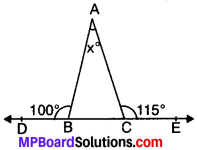To find. x°
∠ABC + ∠ABD = 180° (LPA’s)
∠ABC + 100° = 180°
∠ABC = 180° – 100° = 80°
∠ACB + ∠ACE = 180°
∠ACB + 115° = 180°
∠ACB = 180° – 115° = 65°
Now, ∠A + ∠ABC + ∠ACE = 180° (ASP)
⇒ x° + 80° + 65° = 180°
⇒ x° + 145° = 180°
x° = 180° – 145° = 35°Example 3.
In Fig., PQ ⊥ PS, PQ ∥ SR, ∠SQR = 25° and ∠QRT = 65°.
Solution:
Given
∠SQR = 25°
∠QRT = 65°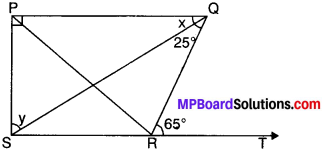To find x and y
In ∆SQR
∠QRT = ∠SQR + ∠QSR (FAP)
⇒ 65° = 25° + ∠QSR
⇒ 65° – 25° = ∠QSR
∠QSR = 40°
PQ ∥ SR and SQ is the transversal.
∴ ∠PQS = ∠QSR (AIA’s)
∴ x = 40°

Example 4.
In Fig., AM ⊥ L BC and AN is the bisector of ∠A. Find the measure of ∠MAN.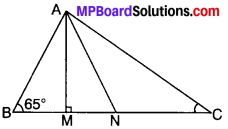Solution:
In ∆AMB
∠ABM + ∠AMB + ∠MAB = 180°
65° + 90° + ∠MAB = 180°
155° + ∠MAB = 180°
∠MAB = 180° – 155° = 25°
In ∆BAC
∠A + ∠B + ∠C = 180° (ASP)
∠A + 65° + 30° = 180°
∠A + 95° = 180°
∠A = 180° – 95° = 85°
∠MAN = ∠BAN – ∠MAB
= $$\frac{1}{2}$$ ∠A – 25°
= $$\frac{85^{\circ}}{2}$$ – 25 = $$\frac{85^{\circ}-50^{\circ}}{2}$$
∠MAN = $$\frac{35^{\circ}}{2}$$ = 17.5°

Example 5.
If the sides of a triangle are produced in order, prove that sum of the exterior angles so formed is equal to four right angles.
Solution: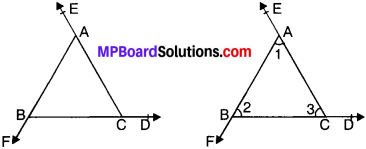∠EAB = ∠2 + ∠3 (EAP) …..(i)
∠FBC = ∠1 + ∠3 (EAP) …..(ii)
∠ACD = ∠1 + ∠2 (EAP) …(iii)
Adding (i), (ii) and (iii), we get
∠EAB + ∠FBC + ∠ACD = ∠2 + ∠3 + ∠1 + ∠3 + ∠1 + ∠2
= 2(∠1 + ∠2 + ∠3) = 2 x 180° = 360°. Proved

Example 6.
Bisectors of angles B and C of a triangle ABC intersect each other at the point O. Prove that ∠BOC = 90° + $$\frac{1}{2}$$ ∠A.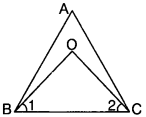Solution:
Given
∠1 = $$\frac{1}{2}$$ ∠ABC
⇒ ∠ABC = 2∠1
∠2 = $$\frac{1}{2}$$ ∠ACB
⇒ ∠ACB = 2∠2
To prove:
∠BOC = 90° + $$\frac{1}{2}$$ ∠A
Proof:
In ∆BOC ∠O + ∠1 + ∠2 = 180°
∠1 + ∠2 = 180° – ∠O …..(i)
In ∆ABC
∠A + ∠ABC + ∠ACB = 180°
∠A + 2∠1 + 2∠2 = 180°
2∠1 + 2∠2 = 180° – ∠A
2(∠1 + ∠2) = 180° – ∠A
∠1 + ∠2 = $$\frac{180^{\circ}-\angle A}{2}$$ = 90° – $$\frac{\angle A}{2}$$ …..(ii)
From (i) and (ii) we get
180° – ∠O = 90° – $$\frac{\angle A}{2}$$
180° – 90° + $$\frac{\angle A}{2}$$ = ∠O
90° + $$\frac{\angle A}{2}$$ = ∠O
∴ ∠BOC = 90° + $$\frac{\angle A}{2}$$Example 7.
In Fig. show that ∠A + ∠B + ∠C + ∠D + ∠E + ∠F = 360°
Solution:
In ∆ACE
∠A + ∠C + ∠E = 180° (ASP) …..(i)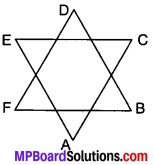In ABDF
∠B + ∠D + ∠F = 180° (ASP) …..(ii)
Adding (i) and (ii), we get
∠A + ∠C + ∠E + ∠B + ∠D + ∠F = 180° + 180°
∠A + ∠B + ∠C + ∠D + ∠E + ∠F = 360°

Example 8.
In Fig., ∠Q > ∠R, PA is the bisector of ∠QPR and PM ⊥ QR. Prove that ∠APM = $$\frac{1}{2}$$ (∠Q – ∠R)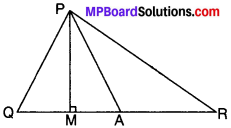Solution:
Given
∠Q > ∠R
∠QPA = ∠RPA
PM ⊥ QR
To prove:
∠APM = $$\frac{1}{2}$$ (∠Q – ∠R)
Proof:
In ∆PMQ
∠Q + ∠QPM + ∠M= 180° (ASP)
∠Q + ∠QPA – ∠APM + 90° = 180°
(∴ ∠QPM = ∠QPA – ∠APM)
∠Q + ∠QPA – ∠APM = 180° – 90°
∠Q + ∠QPA – ∠APM = 90°
∠Q + ∠QPA – ∠APM = 90° …..(i)
In ∆PMR
∠R + ∠RPM + ∠PMR = 180°
∠R + ∠RPA + ∠APM + 90° = 180°
(∴ ∠RPM = ∠RPA + ∠APM)
∠R + ∠QPA + ∠APM = 180° – 90°
(∴ ∠RPA = ∠QPA)
∠R + ∠QPA + ∠APM = 90° …..(ii)
From (i) and (ii), we get
∠Q + ∠QPA – ∠APM = ∠R + ∠QPA + ∠APM
∠Q – ∠R = ∠APM – ∠QPA + ∠QPA + ∠APM
∠Q – ∠R = 2∠APM
∠APM = $$\frac{1}{2}$$ (∠Q – ∠R) Proved

Example 9.
In fig., AB ∥ DC, ∠BDC = 30° and ∠BAD = 80°, find x, y and z.
Solution:
AB ∥ DC and BD is the transversal.
x = 30° (AIA’s)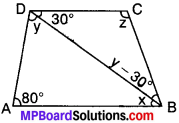In ∆ABD
x + y + 80° = 180° (ASP)
30° + y + 80° = 180°
110° + y = 180°
∴ y = 180° – 110° = 70°
y – 30° = 70 – 30° = 40°
In ∆BCD
30° + 40° + z = 180° (ASP)
[∴ y – 30° = 40°]
70° + z = 180°
∴ z = 180° – 70° = 110°